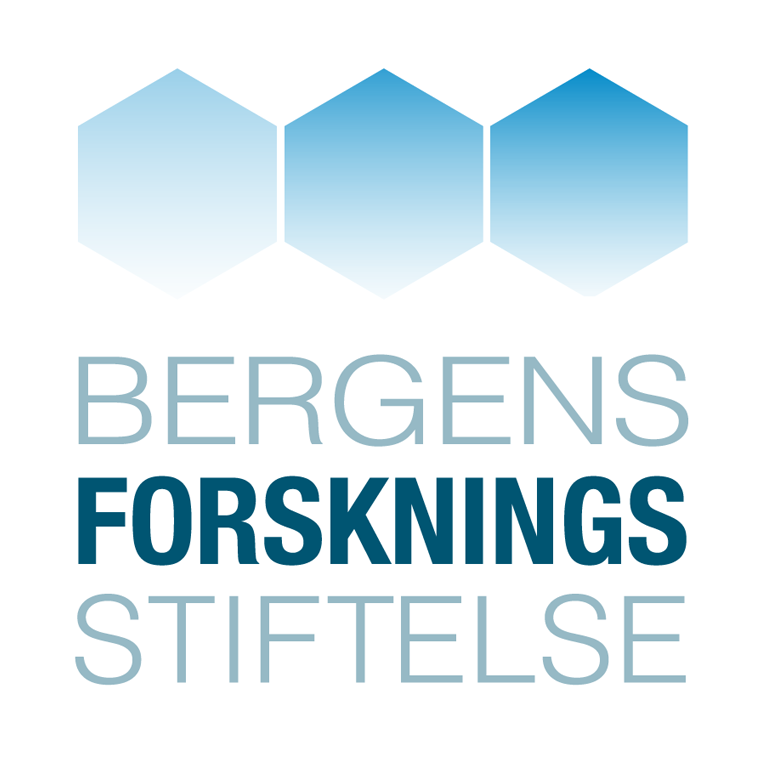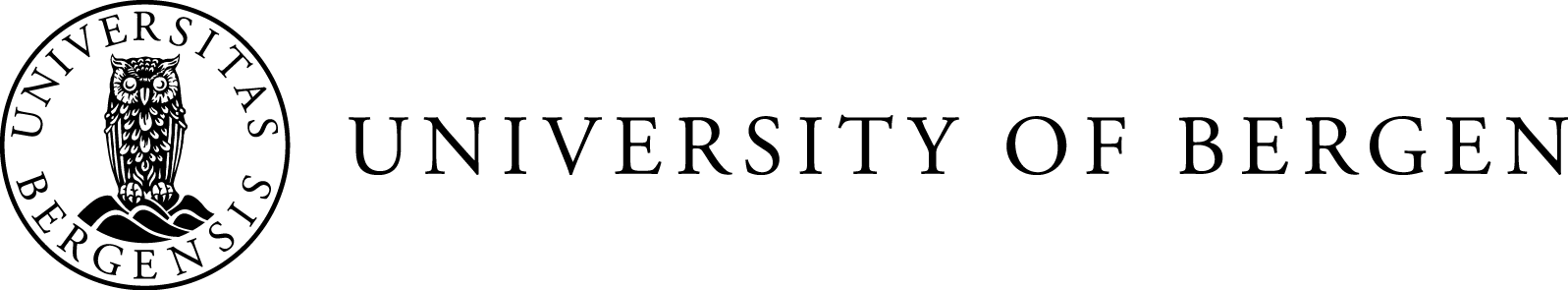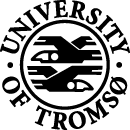## Schedule (pde2019)

Click a talk to display its abstract and presentation.
Main talks are 45 minutes; all other talks 25 minutes.
You may also download the schedule and abstracts as a PDF.

# Wednesday

Download presentation

We will propose the vortex filament equation as a possible toy model for turbulence, in particular because of its striking similarity to the dynamics of non-circular jets. This similarity implies the existence of some type of Talbot effect due to the interaction of non-linear waves that propagate along the filament. Another consequence of this interaction is the existence of a new class of multi-fractal sets that can be seen as a generalization of the graph of Riemann’s non-differentiable function. Theoretical and numerical arguments about the transfer of energy will be also given. This a joint work with V. Banica and F. de la Hoz.

Download presentation

How and why is it so that aggressive cancer cells are able to detach from the primary tumor and migrate to nearby lymphatic vessels? This phenomenon of lymph node metastasis, which is a main reason why cancer becomes a deadly disease, has been recognized for a long time, but is not well understood. Recent experimental work has revealed that interstitial fluid flow can mobilize competing tumor cell migration mechanisms. Researchers have suggested that these different migration modes possibly can play a role in metastatic behavior.

We have formulated a continuum based cell-fluid model where Stokes like momentum equations are used in combination with a transport-reaction system to account for two experimental observed competing tumor cell migration mechanics. We will illustrate basic features of the model. We also briefly indicate how the resulting system of PDEs naturally can be related to current research within multifluid Navier–Stokes models as well as chemotaxis-Stokes systems.

Download presentation

In this talk we will propose a variational method for coherence enhancing image restoration, which is based on a non-convex, non-local integral functional. Because of the separate convexity of the integrand, one obtains lower semi-continuity of the resulting regularisation functional, and, as a consequence, the existence of minimisers. In addition, although the functional as a whole is non-convex, its particular structure allows for the application of exact line search methods, which reduce the chance of converging to local minima. Finally, we will show how the proposed idea is related to PDE based methods based on non-linear transformations of the structure tensor of the images.

Download presentation

In the case of partial differential equations, that govern the motion of waves, classical solutions, in general, do not exist globally. In particular, wave breaking might occur and the solution can be extended to a global one in a non-unique way. In this talk we will motivate how to construct Lipschitz metrics, which measure the distance between two conservative solutions and which reflect the influence of wave breaking on the solution, in the case of the Hunter–Saxton equation.

This is joint work with J. A. Carrillo and H. Holden.

Download presentation

The classical Kolomogorov–Riesz theorem states that a subset of $$L^p$$ is totally bounded if and only if it is bounded, satisfies a tightness property, and is $$L^p$$ equicontinuous. We show that boundedness is not needed, but follows from the other assumptions. Furthermore, we show that this theorem and the classical Arzela–Ascoli theorem both can be derived from a common lemma. This is joint work with H. Hanche‑Olsen and E. Malinnikova.

This takes place in the top floor of the math building (Sentralbygg 2). Elevators are located very close to lecture hall S5.

# Thursday

Download presentation

Mean field game theory was developed since 2006 by J.‑M. Lasry and P.‑L. Lions in order to adapt the concept of Nash equilibria to differential games with infinitely many players. In this context, the value function of any small player depends on the distribution law of the dynamical state of the system. So the mean field model relies on the study of systems of PDEs coupling Hamilton–Jacobi with Fokker–Planck (or continuity) equations. In this talk I will describe several features of mean field game systems and their connection with optimal control and optimal transport, pointing out the role played in this context by weak solutions, renormalized formulations and adjoint methods.

Download presentation

Degrees of freedom is a classical tool for defining finite element spaces. These functionals are used to define the global properties of piecewise polynomial spaces. Furthermore, they can be used to define canonical projection operators onto the discrete spaces which are local and commute with the exterior derivative. However, a fundamental defect of these projections is the lack of boundedness in the natural Sobolev norms. In this talk we will discuss ways to construct alternative projections which have the desired boundedness properties.

Download presentation

The framework of finite element systems was initially introduced to account for the success of some mixed finite elements and extend the techniques to polyhedral meshes and non-polynomial basis functions. In thispresentation we will show how the framework can be generalized, so that it can treat elasticity problems. In particular connections with flat vectorbundles, sheaf theory, and cochain complexes with values in rigid motionswill play a role. In this framework we can define new finite element complexes for prescribed curvature problems. This is joint work with Kaibo Hu.

Download presentation

Recent theoretical and numerical results have shown that inviscid models in gas dynamics, such as the (in)compressible Euler equations, are unstable with respect to initial data or even ill-posed. Going back to the roots of turbulence theory, we interpret instead these hyperbolic conservation laws in a probabilistic manner. We show that statistical solutions of scalar conservation laws are well-posed, and that a weak-strong uniqueness principle holds for general systems of conservation laws. We also study the vanishing viscosity solution under a scaling assumption taken from turbulence theory.

In this talk we want to present a new method for the restoration of well-posedness of singular ODE's in the sense of path-by-path uniqueness of solutions and higher order differentiability of their associated flows via noise. Here path-by-path uniqueness of solutions means that the ODE, which is driven by a merely integrable vector field and a noise given by a fractional Brownian motion with a Hurst parameter $$H<\frac{1}{2}$$, allows for a unique solution in the space of continuous functions $$\mu$$‑a.e. ($$\mu$$ Wiener measure).

We have reserved tables at Hangaren university restaurant, 2 minutes walk from lecture hall S5. Here you can buy food or bring your own food (e.g. sandwiches from the Rapido kiosk).

Lunches are not included in the registration.

Download presentation

I will present joint work with Achenef Tesfahun where we demonstrate ill-posedness of the Maxwell–Dirac system and the Thirring model in one space dimension for initial data which fail to be locally square integrable. These results are sharp, since (global) well-posedness is known to hold for locally square-integrable data.

Download presentation

Pseudo-$$H$$-type Lie groups $$G_{r,s}$$ of signature $$(r,s)$$ are defined via a module action of the Clifford algebra $$Cl_{r,s}$$ on a vector space $$V\cong\mathbb R^{2n}$$. They form a subclass of all 2-step nilpotent Lie groups and based on their algebraic structure they can be equipped with a left-invariant pseudo-Riemannian metric. Let $$N_{r,s}$$ denote the Lie algebra corresponding to $$G_{r,s}$$. A choice of left-invariant vector fields $$\{X_1, \ldots, X_{2n}\}$$ which Lie generate a complement of the center of $$N_{r,s}$$ gives rise to a second order operator $\nabla_{r,s}=(X_1^2 +\ldots+X_n^2)-(X_{n+1}^2 +\ldots+X_{2n}^2 ),$ which we call ultra-hyperbolic. In terms of classical special functions we present families of fundamental solutions of $$\nabla_{r,s}$$ in the case $$r = 0$$, $$s > 0$$ and study their properties. In the case of $$r > 0$$ the operator $$\nabla_{r,s}$$ admits no fundamental solution in the space of tempered distributions. This is a joint work with W. Bauer and A. Froehly (Leibniz Universität Hannover, Germany).

Download presentation

The Brio system is a two-by-two system of conservation laws arising as a simplified model in ideal magnetohydrodynamics. It was found in previous works that the standard theory of hyperbolic conservation laws does not apply to this system since the characteristic fields are not genuinely nonlinear in the whole phase space. As a consequence, certain Riemann problems have no weak solutions in the traditional Lax admissible sense.

It was argued in Hayes and LeFloch (1996 Nonlinearity 9 1547–63) that in order to solve the system, singular solutions containing Dirac delta distributions along the shock waves might have to be used. Solutions of this type were exhibited in Kalisch and Mitrovic (2012 Proc. Edinburgh Math. Soc. 55 711–29) and Sarrico (2015 Russ. J. Math. Phys. 22 518–27), but uniqueness was not obtained.

In this lecture, we introduce a nonlinear change of variables which makes it possible to solve the Riemann problem in the framework of the standard theory of conservation laws. In addition, we develop a criterion which leads to an admissibility condition for singular solutions of the original system, and we show that admissible solutions are unique in this framework.

Download presentation

In the first part of the talk, the physics of the compressible Navier–Stokes equations will be discussed. It turns out that the velocity definition, which in turn is a consequence of the way viscous effects are modelled in a Lagrangian frame, is physically doubtful.

In the second part, a new model for viscous and heat conducting flows is proposed. It is obtained by modelling diffusion, rather than viscosity, in an Eulerian frame.

Download presentation

We consider the initial-value problem for the derivative nonlinear Schrödinger equation (DNLS) on the real line. We implement an infinite iteration of normal form reductions (namely, integration by parts in time) and reformulate a gauge-equivalent equation in terms of an infinite series of multilinear terms. For this new equation we establish estimates without appealing to auxiliary function spaces and thus we show the unconditional uniqueness of solutions to DNLS in an almost end-point space. This is joint work with Haewon Yoon (National Taiwan University).

The dinner is at Radisson Blu Royal Garden, located very close to the Scandic Bakklandet hotel.

# Friday

Download presentation

We show that the compressible Euler system admits infinitely many solutions with prescribed energy profile in the class of Riemann integrable functions. This implies, in particular, the following result: For any given a countable set $$S$$ of times dense in $$(0,T)$$, and any given initial data, the Euler system admits infinitely many weak solutions that are not strongly continuous at any time belonging to $$S$$.

Download presentation

The talk will focus on homogenization problems for convolution type non-local operators with rapidly oscillating periodic or statistically homogeneous coefficients. It will be shown that for a symmetric operator with an integrable kernel under natural ellipticity and moment conditions the homogenization result holds, the limit operator being a second order elliptic operator with constant coefficients. For a non-symmetric operator homogenization result holds in moving coordinates.

Download presentation

To ease the burden of mesh generation in finite-element based simulation pipelines, novel so called unfitted finite element methods have gained much attention in recent years.

In this talk we develop both theoretically and practically a novel cut Discontinuous Galerkin framework (cutDG) by combining stabilization techniques from the cut finite element method with the classical interior penalty discontinuous Galerkin methods for elliptic and hyperbolic problems. The resulting framework allows for the unified numerical treatment of a wide range of problems, including boundary and interface problems as well as surface and mixed-dimensional, coupled surface-bulk and interface-bulk problems.

Download presentation

In this talk we will discuss non-local dispersive equations as models for water waves, and give an overview of recent results on travelling waves for some such equations, in particular on the existence of solitary and highest waves.

Download presentation

It is well-known that viscosity solutions of Hamilton–Jacobi–Bellman equations and entropy solutions of convection-diffusion equations are stable in $$L^\infty$$ and $$L^1$$, respectively. Our aim is to get similar results for viscosity solutions in $$L^1$$ and entropy solutions in $$L^\infty$$. For the first equation, we identify the smallest Banach topology, stronger than $$L^1$$, in which we have stability for viscosity solutions. For the second equation, we propose a new weighted $$L^1$$-contraction principle where we use the solutions of Hamilton–Jacobi–Bellman equations as weights. Interestingly, "any" other weight than the solution of the Hamilton–Jacobi–Bellman equation will give a worse weighted $$L^1$$-contraction principle. This is a joint work with Nathaël Alibaud (UBFC, France) and Espen R. Jakobsen (NTNU, Norway).

We have reserved tables at Hangaren university restaurant, 2 minutes walk from lecture hall S5. Here you can buy food or bring your own food (e.g. sandwiches from the Rapido kiosk).

Lunches are not included in the registration.

## Sponsors (pde2019)

Funded byin collaboration with